Select Page

## Frequency in the Aether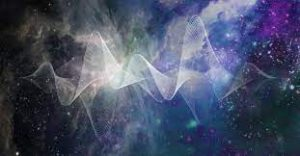As the Phi (φ) angle (137.5o) continually proceeds around a 360o circle, it generates the Phi (φ) spiral, and on a single rotation cycle we can fit two turns of (137.5o) and a remainder of (360o2(137.5o)) = 85o, giving us the ratio for the remainder of 360/85 = φ3. Those two first turns (137.5o) and (137.5o) equal 275, as in 10 times our 27.5 Hz Primal Frequency; therefore, the cubit can be defined as 1” for every 10o in two (2) Phi (137.5o) turns.

You could not use that physically unless you built a circle with a 57.295779” radius, but it is significant metaphysically. The connection to Phi (φ) and Pi (π) merged in the circle is included in every cubit measured out and used in Holy construction, or in divinely inspired construction like the Tower of Truth.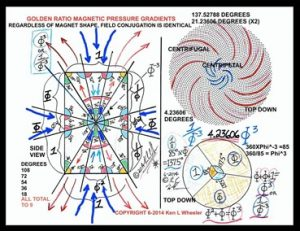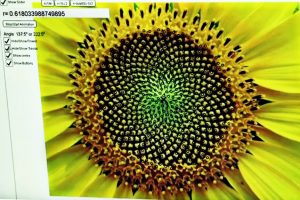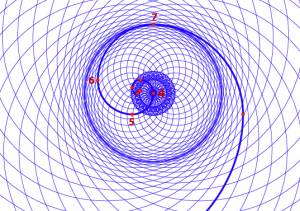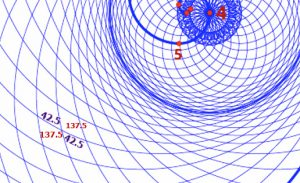The Phi (φ) angles (137.5o) formed by the crossing of the male and female radiant energies also produce counterpart angles of 85o which are equivalent to 137.5o/Phi (φ). This is the same pattern we find in the flowering of the plants and the leaves, branches, and bark of the trees. This is harmony, the perfect balance of the yin and the yang, and through the center flows the life-force, the chi. A pendulum swings between the two forces, the two fixed Phi (φ) angles (137.5o), drawing one then the other. It is 85/2 = 42.5, or Phi (φ)3. It forms an expanding net through the aether.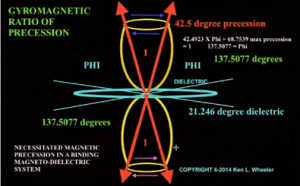A pendulum swings between the two forces, the two fixed Phi(φ) angles (137.5o), drawing one then the other. It is 85/2 = 42.5, or Phi(φ)3, keeping them in balance. It is the gyromagnetic ratio of precession that holds the two equal but incommensurate forces in place. In one 360o revolution, there are 2(137.5)/27.5 = 10 intervals of 27.5 Hz, and since 17 = 27.5/φ and 85 = (5 x 17), there are also 5 intervals of 27.5/φ. As we zero in on the frames of this toroidal spiral to see the precession we get a sense of a non-Euclidean geometry where squares curve into circles, and instead of right 90o angles we have complementary 42.5o and 137.5o ones.

If the Primal Frequency of the aether is 27.5 Hz, and everything is so perfectly symmetrical and fractally oriented why is the temperature in that aether 2.73 K and the Cosmic Harmonic 27.3, and not 2.75? The simple difference between them is (27.5 27.3) = .2, which is .00727272 of 27.5. The inverse of .00727272, which is the difference between them, is precisely 137.5%.

What we are seeing is the interaction between the frequency waves of the aether and the Phi (φ) angular rotation (137.5o). It is all that simple. It is just about peeling back layers. Nothing is hidden from us if we have the desire to roll back our sleeves and seek.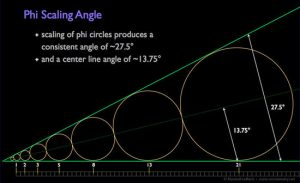Along the Phi (φ) vortex that the Fibonacci Numbers sit on, circles or spheres can be formed that are all in the Phi (φ) proportion to one another, and the angle they lie between is 27.5o. Their mid-line that is drawn through the center of the spheres is 13.75o. Vertically, they represent the Tree-of-Life.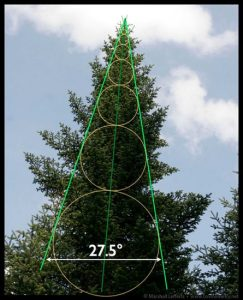The Primal Frequency Radiation emanates from the same Source as the 137.5o Phi (φ) angle and the 42.5o or 42.489o Moshiach Consciousness angle. Since 137.5o/27.5o = 5, every interval of the 137.5o Phi (φ) angle spiral has 4 parallel spirals of 27.5o in between them. They form 5 intervals of 27.5o for each one of the 137.5o Phi (φ) angle ones. Five (5) pulses for each 1 of the Phi (φ) angle ones. In other words, the 137.5o Phi (φ) angle spirals are nothing more than the demarcation of every fifth 27.5o spiral.

As the 27.5o spirals radiate outward centrifugally and centripetally through the aether they too form 42.5o or 42.489o Moshiach Consciousness intersections, and (27.5o +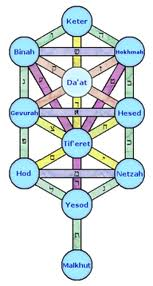42.5o) = 70, as in the 70 Faces of the Torah, the 70 sefirot of the 70 branches of the Tree-of-Life, the 70 processing dimensions of Zeir Anpin and of the Essential Hypercube, the 7 Wells, and the (26 x 70) YHVH (יהוה) in the Torah.

We can now better Understand why the structure of 11 sefirot is called the Tree-of-Life since all trees branch according to the same 137.5o Phi (φ) angle along with the 42.5o or 42.489o Moshiach Consciousness angle, and all resonate to the Primal Frequency (27.5 Hz) as they advance in rings of 27.5o. And “The Tree(הָעֵץ)” of numerical value 165 is (6 x 27.5), the measure of the Holy measuring rod, and the base of the Tower of Truth Sun Triangle.

### Chaos, Phi(φ), and the Primal Frequency

There is yet another connection between the hidden cubit (27.5”) and Phi (φ). It is well known that the division of any two sequential Fibonacci numbers will tend toward the Phi (φ) ratio.  Divakar Viswanath determined that the growth rate of the random recurrent Fibonacci sequence is a constant equal to 1.1319882487943, which is known as Viswanath’s constant.  Alas, since he knew nothing about the cubit or Primal Frequency (27.5) he missed the connection between his constant, Phi (φ), and the cubit.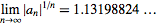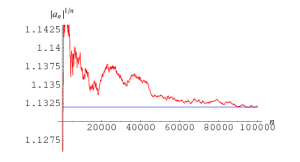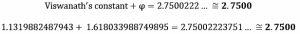# The 27.5 HZ Frequency and Tesla

Applying Modulus mathematics to the Fibonacci Numbers yields all sorts of fascinating results with cyclical repeats. To us what is most fascinating of all, happens when we apply mod (27.5) to the Fibonacci numbers to get a cyclical repeat cycle of 20, meaning every 20 Fibonacci numbers the cycle will repeat exactly, and their sum is exactly 165, a natural cosmic alignment with the base of the Tower of Truth and Sun Triangle and its 20 sequential stages of stacked cubes height.  It is also the measure of the rod, 165 inches.Of course, 165 is the small gematria0 of the 42-Letter Name, the same version used in 9/.1230490273… = 73.14157771495, and at digit #165 in Pi (π) we find the string …2701… as in the Torah’s first verse, the 73rd Triangular Number.Everything derives from the Source.

Applying quotient (27.5) to the Fibonacci Numbers produces yet another cycle. The remainder/whole number is #10 for every 10 numbers similar to the set of all integers, but this is counterintuitive as the Fibonacci Numbers, while sequential are not consecutive, nor evenly spaced apart.  In fact, their spacing accelerates according to the Phi (φ) proportion. And while that spacing is proportionately ever accelerating the ratio between the resultant whole number interval values increases by exactly 123 for ever 10th Fibonacci Number, which works out to Phi (φ)10, as in the first 3 Letters and digits in the 42-Letter Name (אבג). The sum of the integers from (110) = 55, and every 10th Fibonacci Number is a multiple of 55 and 123, the while the mod (27.5) cyclical sum of 165 is (3 x 55).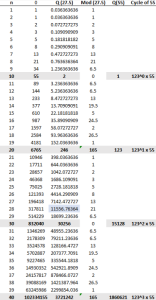The relationship between Phi (φ), the Primal Frequency (27.5) and the concept of 10 is ever more profound and ever more symmetrically beautiful.

What also stands out is the universe’s relation to the Spherical Time radius 5778 or Phi (φ)18 through a string of synchronous associative numbers. In the spreadsheet above we see that the 28th Fibonacci Number divided by the Primal Frequency (27.5) equals 11,556, which is the Spherical Time Bubble diameter and twice 5778, and 5778 is the 107th Triangular Number, while 107 is the 28th Prime Number.

# The 27.5 HZ Frequency Determines the Speed of Light

In our physics, a frequency is determined by the distance between two wave peaks and the higher the frequency the closer together those peaks and thus the shorter the distance between them and shorter their wavelength. That pretty much defines the entire electromagnet scale as we understand it. If 27.5 was a frequency, say 27.5 Hz, its corresponding wavelength in space solely contingent on the speed of light, would be 6773.90539 miles. A single wavelength would take up most of the diameter of the Earth. The numeric combination of the Binah (67) and Chochma (73) values is easily recognizable in 6773, but this could be entirely coincidental. The speed of light divided by 1 cubit, or the Primal Frequency (27.5 Hz) is 186,282 mps/27.5 = 6773.9 miles.  What is not coincidental is that 6773.90539 miles is certainly 6774 miles in whole numbers and that the sum of the 10 square roots of the resultant 10 remainders of dividing by (27.5), from .090909 to .909090, is 6.774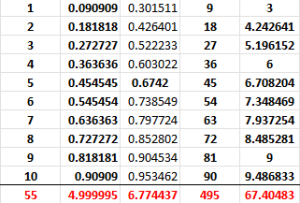The sum of the results of the 10 square roots of those 10 resultant remainders, as in .090909…, .181818…,.272727….909090, is 6.774…, matching the value for the wavelength of 27.5 Hz against the speed of light, 6774 miles.

There should be no logical correlation between them, unless those 10 residues of quotient (27.5) relate to the speed of light, which makes no scientific sense, unless of course the universe was intentionally designed that way. That would mean that the speed of light might be dependent on the radiance of the Primal Frequency in the aether, and that the physical limitations imposed by the speed-of-light might just be a matter of fine-tuning.

Now, the sum of the square roots of the 10 cyclical remainders of the 27.5 application is 6.774, which equals the wavelength (6774 miles) of the Primal Frequency (27.5 Hz), and when we use the Pi (π) gematria cipher to calculate the value of the 31 initial words of the 31 verses of the Torah’s first chapter, they equal 6774.The constant 27.5 is pretty special.  There is almost no aspect of our modern physics that is not dependent on the constant (c) as the speed of light. And yet, the universe was created so that it was based not on propagating waves or imaginary particles but on a single simple number, 27.5, and its intersection with the simplest of base 10 mathematics, what we have previously studied as the 10 fields from 1 to 10.

As we just saw, the speed of light, 186,282 mps (miles per second), ties the sacred cubit/Primal Frequency (27.5) to the essence of the number 9 as delineated by the 10 sefirot (dimensions). This is the same sacred cubit that is dedicated to being used in the building of the Future Holy Temple. It is the same concept of frequency at the core of Spherical Time and throughout the aether.

The Moon’s circumference, 6782 miles, is about (67826774) = 8 miles off the exact wavelength of the 27.5 Hz Primal Frequency, the frequency of 1 cubit, 6774 miles.

The Earth’s diameter varies according to where it is measured from 7900 miles at the poles to 7926 miles at the equator. At the average standard 7920 miles, its circumference is 24881 miles, as in the year Mosesdied and the Israelites entered Israel (2488 HC), which is 1273 years before 3760, and is in perfect alignment with the cosmic harmonic 1.273.  The Moon’s circumference on the other hand is only 8 miles larger than 6774 wavelength of 27.5 Hz.

The difference between the diameters of the Earth and the Moon is (79202160) = 5760 miles, analogous the year 2000 CE (5760 HC) and aligned with log (5760) that equals 3.760 or 2.000 less than 5.760.

At 27.5 Hz, the corresponding wavelength in space, meaning a wavelength solely contingent on the speed of light, is 6774 miles, or 67.20% of the Earth and Moon diameters together (10080 mi).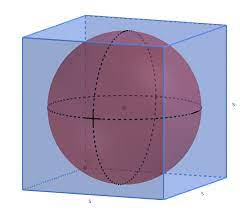Using the equatorial diameter, the Earth’s circumference is thus 3.676 wavelengths of 27.5 Hz, and its inverse is 1/3.676 = .272022, which is exceeding close to 2.72027, the ratio of the volume of the inscribed cube to the Sphere, especially given the variation in the Earth’s diameter.

Thus, the Moon is a sphere approximately equal to the wavelength of Primal Frequency (27.5 Hz), and the Earth is a sphere that circumscribes the moon as if were a cube.

The Sun has a circumference of 2,720,984 miles or roughly 2.72027 times 1 million (106) miles. The Sun is a sphere whose size is 1 million times the hyperspace (metaphysical) ratio between the Earth and the Moon.

One million (106) is a 6-fold expansion of 10, as in the 10 sefirot (dimensions), a design that strictly corresponds to the 6 folded dimensions of string theory (the Calabi–Yau manifold) and the 6 bundled dimensions of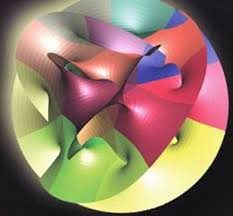Zeir Anpin, which is what the ancient Kabbalists have always said that the Sun represents.

For the metric enthusiasts, there is also the simple cosmic field equation (1/φ – 1/π) = .2997241.. which is off from the perceived speed of light, 299,792,458 m/s by only 48 m/s, which we can then couple with another simple equation derived from Spherical Time, 36304.24470 = 27.52 x 48.00561… as in the 480,000-mile diameter of the lunar orbit, and the 48 stories of the Tower of Truth and Pyramid of the Sun, especially since 27.52 x 48 = 36300 inches or 4.24470 less than the Spherical Time circumference, and since 36300 inches is the perimeter of said Pyramid.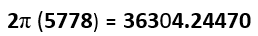Twice those 6774 miles or 2 wavelengths of 27.5 Hz is 13,548, which is 1998 or (3 x 666) more than twice the height of the Tower of Truth and the resultant Pyramid of the Sun or (2 x 5775”) that is also 420 cubits. Or (6774 5775) = 999 = (3 x 333).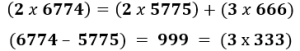Meanwhile, the cube root of the Spherical Time circumference, 36304.24470 is 33.1120 as in 33 and the 112 Triplets.

# The 10 Remainders and the Tree-of-Life

All 10 remainders (.090909…, .181818…, .272727…, .363636…, .454545…, .545454…, .636363…, .727272…, .818181… and .909090…) were derived from a single cubit (27.5) or single frequency.  The pattern is a simple 10 remainders, a whole number, the same 10 remainders, the next whole number…, 10 out of every 11 integers, all processed in quotient (27.5). Hyper-dimensionally they can be seen as the 10 sefirot (dimensions) out of every 11 sefirot.

Think of them as a string of lights and every 11th is turned off.  The 10 bright bulbs represent the complete set of 10 complementary infinite remainders based on the number 9. They always add up to 5.  The 11th number will always be a simple finite number.Separating the whole number as representative of the dimension of Keter, the way the Torah does with the 10 Commandments, etc. the 10 remainders align with the 10 sefirot from Chochma to Malchut.

Since the actual repeated pattern is .363636…, .727272…, .909090…, .454545…, .818181…, .181818…, .545454…, .090909…, .272727…, and .636363… these 10 remainders can be split just like the Tree-of-Life into their top 3 and bottom 7 identities, and they split into totals of precisely 2.0000 for the top 3 and 3.0000 for the bottom 7 of Zeir Anpin, a 40/60% split.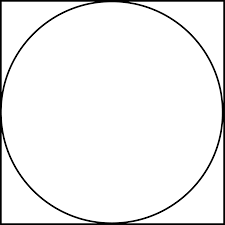Of that total, Malchut, the level of physicality, represents 12.73%, as in the Cosmic Harmonic 1.273, and the square that circumscribes the circle.

The metaphor is simple: Like the Earth and Moon symbiosis enveloping the Earth, Physicality envelops and boxes in Spirituality.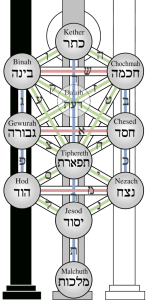Those 10 remainders are determined by the frequency 27.5 Hz. Those 10 remainders’ sum is always 5, and their product is always .00014, as in the central position of the Magical Essential Cube of Creation and of the Spherical Time paradigm.

The inverse of their product is 7147.73 and 7174.73 minus the wavelength of 27.5 Hz or 6774 miles is (7147.736774) = 373.73037…, as in the equation (37 x 73) = 2701, representing the Torah’s first verse, and the inner (37) and outer (73) Light (gematria values) of the sefira (sphere) of Chochma (חכמה), the 9th sefira, or highest attainable one.

### The Torah Paragraphs and Phi (φ)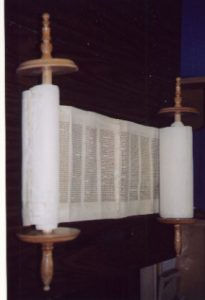The 373 comparative result is also aligned with the 37.3% of the 670 Paragraphs in the Torah that are contained in the first (91) and last (159) Books, leaving 420 in the other central 3 Books. The 670 paragraphs are in turn aligned with the level of Binah (בינה) of numerical value 67.

Chochma (חכמה) and Binah (בינה) together equal (73 + 67) = 140, as in the area covered by the first 7 perfect squares (12 + 22 +…72) = 140, representing the 7 dimensions beneath these upper two, and the value 140 too is found among the 72 Triplets (עלם), as the 4th one. Its sofit value is 700.

The first Book of the Torah, Bereshit (Genesis) has 91 Paragraphs, connecting it to the Path of One, meaning 86.42% of the Paragraphs are in the last 4 Books. Nonetheless, of those 420 central Paragraphs, the 256, or 28 Paragraphs, in the Books Vayikra (Leviticus) and Shemot (Exodus) together split the 670 total into the exact Phi (φ) proportion (256/670). Then those two portions are in turn split again (98 + 158) in almost exactly the same Phi (φ) proportion (98/158).

The value or area covered by 91 also represents the first 6 perfect squares (12 + 22 +…62) = 91, representing the 6 dimensions of Zeir Anpin, and it too is found among the 72 Triplets (סאל), as the 45th one.

### Splitting of the Red Sea

There are 91 paragraphs in the first Book of the Torah, and 39 more up until the Splitting of the Red Sea, or Endless Sea, in Paragraph 40, chapter 14 of the Book of Shemot (Names) that is also known as Exodus.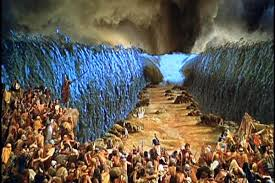This means that the Splitting of the Sea also splits the Torah into 130 Paragraphs and 540 paragraphs, as in the value of Mt Sinai (130) and the value of the 57th Triplet (נמם) of the 72 Triplets. The value 540 on the other hand is associated with the other Cosmic Mountain, the Pyramid of the Sun, whose height and base equal (210 + 330) = 540, and with the first 6 digits, the first two Triplets, in Pi (π) whose product is (3 x 1 x 4 x 1 x 5 x 9) = 540.

The Splitting of the Sea consists of 3 consecutive verses of 72 letters each that are provably derived from the 72 Name (Triplet) Matrix. As the 72 Triplets unwind, their 72 initials become the 72 sequential letters of 14:19, and their 72 final letters become the 72 sequential letters of 14:21. The first verse of 72 or 72 initials total 2672, as in the 26 value of the YHVH (יהוה) and its 72 value of its spelled-out version (יוד־הי־ויו־הי). It is also aligned with the Pyramid of the Sun’s apothem, 267. The final letter verse of 72 has a value of 3428, leaving the difference between the two verses (34282672) = 756, the base measure of that same Pyramid. The middle verse comes directly out of the middle 72 letters in the Triplets except in their reverse order.

### 21 And Moses stretched out his hand over the sea; and the LORD caused the sea to go back by a strong east wind all the night, and made the sea dry land, and the waters were divided.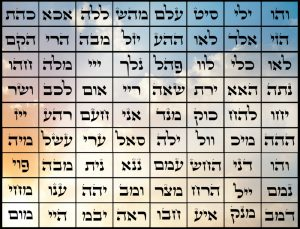We have already highlighted numerous Triplets that directly reflect the values of the cosmically and geometrically derived Pyramid of the Sun and the there are many more. Then there are some that reflect the values of certain key Names, like 25th Triplet (נתה) that has a value of 455, corresponding to the 3 Aspects of the higher Name Ehyeh (אהיה), and when added to its ordinal position 25, gives us the height of the Sun Pyramid in feet (480). There is also the last of the 72 Triplets (מום) that has the value 86, as in Elohim (אלהים), and the 5th one (מהש) that has the value and permuted letters of Moses (משה), 345. We can easily connect most of them to specific Names and aspects of Names, like the two twin Triplets (לוו) and (וול) of value 42 and the 5 other Triplets that have the MB (מב) or 42 and who’s accompanying 5 letters spelled the name Yehuda (יהודה) found 42 times in the Torah. Or simply like the 22 and 27 letters that correspond to the 7th (אכא) and 40th (ייז) Triplets, etc., etc. Nonetheless, this shows the complexity and symmetry throughout the cosmic elements yet does not prove that the Triplets predated the Torah despite the statistically astronomical odds of this occurring by random chance.

The proof, though, is found in the perfection of the square roots of their Triplet values. It not only proves the Matrix was always designed to be in this exact order and presentation but because the letters in the Matrix change form to their final or sofit shapes as necessary when transposed into the 3 verses, they had to precede them. It is a one-way transformation.

The sum of the average individual Triplet square roots for all 72 Triplets is 720.0 and the sum of the square roots of the totals for the 9 rows taken individually is 280.0, while the same equivalent for the 8 columns is 267.65 as in the Sun Pyramid Apothem once again.  Together they equal 1000.0, an astonishing result, like the 1000 average of each column and each row in the 8 x 8 Foundation Stone square formed by the Matrix’ bottom 8 rows.

We can separate out the first row that totals 1143, from the total 9143 value of the Matrix because 1143 is also the average of the 8 columns (9143/8) = 1143, and the average value of the Triplets in that first row is (1143/8) = 143.

It is a perfect and beautiful symmetric design infinitely more complex than any normal Magic square, yet it gets better. The sum of the average individual Triplet square roots for the 9 rows is 90.00, and the sum of the average individual Triplet square roots for the 8 columns is 80.00, while the average square root for the 72 Triplets is 10.000.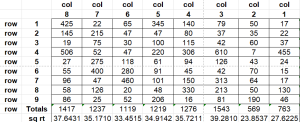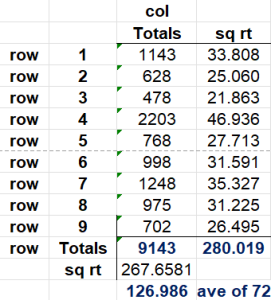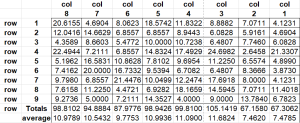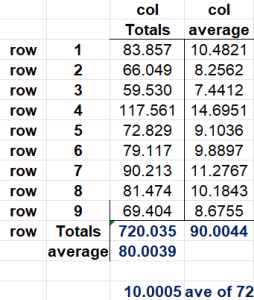There is so much more technology embedded in this Matrix that predates the Torah, like the central 4 letters (הרדא) of the Matrix that equal 210, as in the height of the Sun Pyramid and the 210 years of exile in Egypt, and the 4 initials (שרמא) of those 4 central Names that add up to 541, the value of Israel.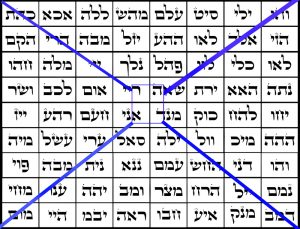### A Perfect Split

Whether the Creator is just playing with our minds, or leaving breadcrumbs so we will dig deeper, He made the average for the 72 values approximately 127, as in the age of Sarah prominently highlighted in the Torah and as in the 7th Mersenne Prime that helps determine the 4th Perfect Number, 8128, which is 872 less than 9000, reflecting the 8 rows and 9 columns of the 72 Triplet Matrix.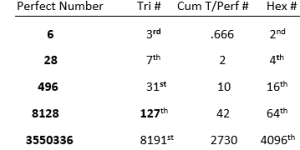And yet, the first word in the Torah has 6 letters like the first Perfect Number, and the first verse has 28 letters like the second Perfect Number. Moreover, the 7 words of that verse can recombine in exactly 127 possible ways with each of those words utilized exactly 64 times, in the same way that the word value 127 is utilized exactly 64 times in the Torah, aligning them with the associated 127 and 64 ranks of the Triangular (8128) and Hexagonal Numbers (8128) that compose (127 x 64) = 8128, the 4th Perfect Number, as in (4 x 7) = 28, and as in the 64 square Foundation Stone within the 72 Triplet Matrix.

As for the other two Perfect Numbers before they become two large to manage, 496, the third Perfect Number is the 31st Triangular Number, which is twice 248, which itself is (8 x 31) and which represents Abraham, whose Name is found concealed in the first verse. While the sum of the first 8 initials of the 10 sefirot equal 496, that of the Name of the last sefira, Malchut (496), 127 is the 31st Prime Number.

Meanwhile, the sum of 8 of the first 10 Triplets in Pi, sum to 3550, as in the fifth Perfect Number 3550336 that may also include a reference to the 336 letters of the 112 Triplets, and to the 3550 Men counted in the census whose half-shekels were temporarily misplaced.

# The Inverses of the 10 Remainders

The inverse of the 4th remainder of those 10 Primal Remainders is 1/.363636 = 2.75000… simply put, it equals the sacred cubit (27.5 inches) and Primal Frequency (27.5 Hz). Pretty fitting, since it is the residue most structurally connected to 3, 6, 9, and to 363, the repeated gematria of H’Moshiach, the Cosmic Consciousness.

Thus, we see that the sacred cubit and Primal Frequency (27.5) and H’Moshiach (363) Consciousness have an inverse relationship, and thus when multiplied together (27.5 x .3636363), they equal One (1).

The inverses of the 1st, 2nd, 5th, and 9th remainders are respectively, 11.0000, 5.50000, 2.20000, and 1.10000, all reflective of those numbers’ derivative relationships with quotient (27.5) that we previously discussed. We could even say that they must be derivative of the 4th remainder that we just highlighted, 2.75.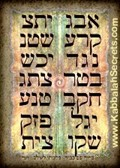The inverse of the 6th remainder, 1/.545454 = 1.833333…, analogous to both the midpoint of the 42-Letter Name (1833) and the 1833 letters Samechs (ס) of numerical value 60 found in the Torah.

The inverse of the 8th remainder, 1/.727272 = 1.37500…, which is analogous to the 50-cubit height (1375”) of the 10 Gates of the Holy Temple and of Noach’s Ark, and of the 3 final levels of the 42-Letter Name Matrix.

The inverse of the 7th remainder, 1/.636363 = 1.571430, and while 1571 is the 248th prime number, it is also one-half of 3.142860.  The value 3142 has been covered repeatedly already as the small gematria of “I am that I am (אהיה־אשר־אהיה)” found at Exodus 3:14 and the numerical value of the 3 iterations of the Names Ehyeh (אהיה) and YHVH (יהוה).It is also built into the Tower of Truth/Triangle of the Sun perimeter. It is also the difference (3.1428) between the atomic weights of gold, silver, and copper (368.3988) that are associated with the central 2 Triplets of the 72 Triplets, and the sidereal year, 365.256 or (368.3988365.256) = 3.1438.

We find it again appropriately as 7.3142, the result of dividing the 42-Letter Name Matrix by its 1st level, 3701/506 = 7.31422, and again by dividing 9 by that same first level in small gematria, 9/.123049 = 73.142, or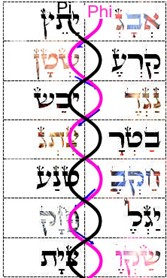more accurately, 73.141593.

The value 506 first presents itself in the Torah within its first verse as the difference between the values of its first word and its sixth, between its highest word value and its next highest (913 407) = 506. The value or area covered by 506 also represents the first 11 perfect squares (12 + 22 +…112) = 506, representing the 11 dimensions of the Tree-of-life. Counting from the bottom of the Tower of Truth, the area covered through that 11th stage is 2485, the 70th Triangular Number, as in 70 + Pi (π).

The value 506 is also found amongst the 72 Triplets, in the 32nd Triplet (ושר) with the value 32 representing the 32 Paths that connect the 11 Sefirot of the Tree-of-Life.

### 11 Year Cycle

By dividing numbers by the value of the frequency (27.5 Hz) the Primal Frequency becomes a filter that sorts them into groupings of 10, whose remainders are all based on 9: .090909…, .181818…, .272727…, .363636…, .454545…, .545454…, .636363…, .727272…, .818181… and .909090… between every 11th marker number.

Those markers (numbers) are in cycles of 11, like our 11-year solar cycle, starting with 11, 22, 33, 44…. Each cycle advances by the result (11/27.5) or .4, as in (.4, .8, .12, .16…) for each consecutive marker. The number 110 is the 10th cycle of 11 years/numbers and has a resultant value of exactly 4, as in (10 x .4) and as in (4 x 27.5) = 110.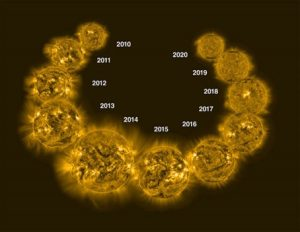As for the 10 bundled numbers within each set of 11, the sum of the results of dividing the consecutive integers by 27.5, starts with 2 for the first set of 10 and advances 4 integers (units) with every subsequent set (2, 6, 10, 14, 18…). We see it as a continual series of mathematical operations, but to the universe it is a coded program.

 1 0.036364 1 0.036364 2 0.072727 2 0.072727 3 0.109091 3 0.109091 4 0.145455 4 0.145455 5 0.181818 5 0.181818 6 0.218182 6 0.218182 7 0.254545 7 0.254545 8 0.290909 8 0.290909 9 0.327273 9 0.327273 10 0.363636 10 0.363636 11 0.4 55 2

The first observation is that there is an affinity for the frequency 27.5 and for the number 4 in bundles of 10, not to mention cycles of 11, in a similar way that the 4th Triangular Number is (1 + 2 + 3 + 4) = 10.

Naturally, the first set of (110) integers (units) = 55 and then when divided by the sum of the remainders we get 55/2 = 27.5, which aligns with the average value of the 2 Heis (הה) of the 4 letter YHVH (יהוה).

 12 0.436364 12 0.436364 13 0.472727 13 0.472727 14 0.509091 14 0.509091 15 0.545455 15 0.545455 16 0.581818 16 0.581818 17 0.618182 17 0.618182 18 0.654545 18 0.654545 19 0.690909 19 0.690909 20 0.727273 20 0.727273 21 0.763636 21 0.763636 22 0.8 165 6

The second set of 10 integers from 1221 total 165, as in the base (165 cu) of the Tower of Truth Triangle. Its 10 remainders total 6, so once again get 165/6 = 27.5.

 23 0.836364 23 0.836364 24 0.872727 24 0.872727 25 0.909091 25 0.909091 26 0.945455 26 0.945455 27 0.981818 27 0.981818 28 1.01818 28 1.01818 29 1.05455 29 1.05455 30 1.09091 30 1.09091 31 1.12727 31 1.12727 32 1.16364 32 1.16364 33 1.2 275 10

The third set of 10 integers from 2332 total 275, as in Primal Frequency, and with its remainders totaling 10 we once again get the constant ratio 275/10 = 27.5.  The first set of patterns are clear. The system is entirely dependent on the Primal Frequency 27.5, and while every 11th whole number result in quotient (27.5) advances by .4, every 10 remainders as a sum advance by 4.

### The First Inflection Point

The 5th bundled set of 10 from (45 – 54) adds up to 495. This aligns with the first 18 letters of the Alef-bet, which equals 495. It also aligns with the 495 rotations over the 25,920-year Precession Great Cycle and to the 495o cycles that equal 2.75π radians. It also aligns with the 3 x 3, 6 x 6, and 9 x 9 Magic Squares, which together equal (15 + 111 + 369) = 495, and to the 495o turned in Spherical Time every 10 seconds because of Phi (φ).

Fittingly, since 495/18 = 27.5, this 5th interval or set has a 27.5-cycle value of exactly 18.

 45 1.63636 46 1.67273 47 1.70909 48 1.74545 49 1.78182 50 1.81818 51 1.85455 52 1.89091 53 1.92727 54 1.96364 495 18

Moreover, all 50 (5 x 10) bundled numbers in the first 5 intervals, which corresponds to the 55 (5 x 11) total numbers, have a 27.5-cycle or quotient value of exactly (2 + 6 + 10 + 14 + 18) = 50.The quotients are determined by dividing by 27.5; nevertheless, the 50 cycles that are determined by multiplying is also special because (50 x 27.5) = 1375, as in the Phi (φ) angle (137.5o) and as in the final 3 rows of the 42-Letter Name Matrix that equal 1375. It is also the sum of the first 5 intervals (55 + 165 + 275 + 385 + 495) = 1375, and the height of the Gates in the Holy Temples (1375”) etc. We need to ask ourselves why the Creator would design the Holy Temples with Gates that are 50 cubits tall when few men are even 3 cubits tall? Why must the Gates be at a height that matches the angle of Divine Radiation (137.5o)? And why must the walls of the Holy Temple be exactly 6 cubits or 13.75 ft thick? Does the Light that is generated through the Holy Temple resonant at this frequency?This 5th interval is a very specific inflection point in the scale of all integers divided by 27.5.

As the integers increase, their resultant quotients total closer and closer to the number of the bundled results until the number 50, corresponding to the 54th integer and the interval at 55. The first bundled set of 10 results equals 2, the 2nd or 20 results equals 8, the 3rd or 30 results equals 18, the 4th or 40 results equals 32, and the 5th or 50 results is exactly 50. From here, though, the relative values get further out of alignment as the first 6 bundles, or 60 results equals 72 and the first 7 bundles or 70 equals 98.

Thus, at the 54th integer there are 5 bundles of 10 completed or 50 in total and the sum of the 50 bundled results is exactly 50. Beyond 50, the cumulative sum of the quotients grows larger and further away from the number of bundled results. Hence 50 is the unique inflection point for the bundled results, like the limit of the 50 Gates of Binah.

 32 1.16364 29.0909 1.16364 19.2 33 1.2 30 18 20.4 34 1.23636 30.9091 1.23636 21.6364 35 1.27273 31.8182 1.27273 22.9091 36 1.30909 32.7273 1.30909 24.2182 37 1.34546 33.6364 1.34546 25.5636 38 1.38182 34.5455 1.38182 26.9455 39 1.41818 35.4545 1.41818 28.3636 40 1.45454 36.3636 1.45454 29.8182 41 1.49091 37.2727 1.49091 31.3091 42 1.52727 38.1818 1.52727 32.8364 43 1.56364 39.0909 1.56364 34.4 44 1.6 40 32 36 45 1.63636 40.9091 1.63636 37.6364 46 1.67273 41.8182 1.67273 39.3091 47 1.70909 42.7273 1.70909 41.0182 48 1.74545 43.6364 1.74545 42.7636 49 1.78182 44.5455 1.78182 44.5455 50 1.81818 45.4545 1.81818 46.3636 51 1.85455 46.3636 1.85455 48.2182 52 1.89091 47.2727 1.89091 50.1091 53 1.92727 48.1818 1.92727 52.0364 54 1.96364 49.0909 1.96364 54 55 2 50 50 56 56 2.03636 50.9091 2.03636 58.0364 57 2.07273 51.8182 2.07273 60.1091 58 2.10909 52.7273 2.10909 62.2182 59 2.14546 53.6364 2.14546 64.3636 60 2.18182 54.5455 2.18182 66.5455 61 2.21818 55.4545 2.21818 68.7636 62 2.25454 56.3636 2.25454 71.0182 63 2.29091 57.2727 2.29091 73.3091 64 2.32727 58.1818 2.32727 75.6364 65 2.36364 59.0909 2.36364 78 66 2.4 60 72 80.4 67 2.43636 60.9091 2.43636 82.8364 68 2.47273 61.8182 2.47273 85.3091

The inflection point at the number 50 is the sum of the 50 bundled remainders, through 54 or 55, yet the inflection point at the number 54 is also the total sum of ALL 54 integers in quotient (27.5).

As the numbers get closer to this inflection point at integer 54, the sum of the previous remainders gets closer but never equal to the integer value until number 54. Then for every integer above this inflection point of 54, the value of the collective bundled results gets higher and higher vis-à-vis the number related to them.

The last or 5th set of 10 remainders through 54 totals (5032) = 18, or 1/3 of 54, as seen in the chart provided. This unique inflection not only aligns with the 54 Portions of the Torah, but also with the remainder of the extraordinary sum of the 72 square roots of the 72 Triplet Matrix and the 9 square roots of its 9 rows, 1000.054.

Meanwhile, the first 3 of the 5 sets also total 18. This 18-total value occurs at the 33rd integer, once again tying 18 and 33 together, as we saw in the exact center of the 42-Letter Name that totals 1833.  Of course, if the first 3 sets of 5 equal 18 and the last set of the 5 equals 18, then the 4th set must equal (3618) = 18 as well, totaling 54.

Since the 55th integer is ostensibly the same inflection point as 54, it represents the 5th interval.

That 5 bundles of 10 should equal 50 is special, and that it is an inflection point makes it extraordinary, connecting them to the 50 Gates of Binah and to the 10 Gates of 50 cubits height and 10 cubits width each in the Future Holy Temple.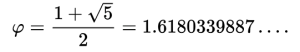We know that the number 5 is at the core of the Torah and of Phi (φ) and of 27.5, as both (5 x 5.5) and the limit (5.0) to each bundle of 10 of the 27.5-cycle (quotient 27.5) results or residues.

We also know that we are talking about the set of all numbers taken sequentially, one decimal place at a time in the base-10 system of mathematics. We just saw the connection between Pi (π) and 18 and the 3142 value of the highest Names of the Creator associated with Binah. The number 50 keeps coming up and it too continually relates to the 50 Gates of the hyperspace dimension of Binah. It all bears keeping in mind, especially the part that the 11-number cycle plays in all this.

Scientists are still perplexed why by all observations the Sun conforms perfectly to an 11-year cycle of activity. The 11-year solar cycle starts off with a flipping in the Sun’s magnetic field.  The Sun’s polarity switches from N to S and S to N every 11 years. This kicks off a Solar Minimum with few sunspots and coronal emanations. Over the 11-year cycle, those sunspots, coronal emanations, and solar flaring build to a Solar Maximum halfway (5.5 years) through the cycle before waning again towards the next cycle. That is its inflection point.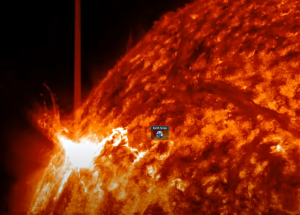Just last week, one of those sunspots erupted in a super flare. Fortunately it was facing away from the earth, If it has been 9 days all civilization on the planet would have been wiped out in short order. It was not the predicted and prophesied 11,556 year Spherical Time cyclical one that will be significantly more powerful and align with the ever accelerating degradation of the Earth’s magnetic fields. It was just a somewhat typical element to the cyclical solar maximum. We were fortunate; had it struck Earth, every electronic device and every power grid would have shorted out and fried, resulting in fires and explosions and with no way to address them. Was it a warning? Perhaps. Most people who took notice took it as an opportunity to see more beautiful auroral northern light displays.

Sunspots revolve around the Sun in exactly 27.3 days, the Cosmic Harmonic, so there is a 50/50 chance that when a large one fires off a mass ejection that it will be pointing in the general direction of Earth, maybe a little less depending in what direction it fires. This is the same 27.3 days that our Moon orbits the Earth, which is the same 27.3 days that it rotates around with respect to the stars.  One fluid motion, all magnetically aligned. This is 13.378 times every solar earth year and 147 times in 11 years, as in the 147 years of Jacob’s life, for both the Moon and the Sun.

Some maximums are more powerful than others and their effect on Earth is more profound, yet the cycle never changes, like clockwork.  Given the state of technological dependence on Earth, a very powerful solar maximum could have devastating consequences for humanity, and yet just be part of the Sun’s natural cycle.

# The Sacred Heartbeat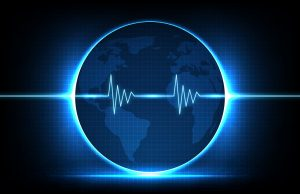There is a second Primal Frequency (1.1 Hz), but as 1.0% of 110 it is really a derivative of 27.5 Hz, yet since it is connected to 11 it has very interesting interactions with 27.5 Hz. These become readily apparent when the two sets are lined-up beside one another.

The Primal Frequency (27.5 Hz) that gives us a heartbeat of 11 breaths or beats, as in the Vav-Hei (וה) of the YHVH (יהוה) and of the Sun’s cycle, is aligned with the pulse of 11 in yet another way besides the set of 10 of every 11 integers repetition.

When we divide the set of all numbers by 27.5, it gives us groupings of 10 of every 11 numbers with remainders in groupings of 9’s: .363636…, .727272…, .090909…, …,.454545…, .818181…, .181818…, .545454…, .909090…, .272727…, .636363….

If instead, we divide the numbers by 1.1 or by any multiple, such as 11, the groupings also similarly and parallelly form in sets of 10 out of each 11 integers, but this time the remainders are all in sequential order from lowest to highest: .090909…, .181818…, .272727…, .363636…, .454545…, .545454…, .636363…, .727272…, .818181… and .909090.

The first group collectively sums in quotient (1.1) to 50, as in Binah, with the set of every 11th integer increasing by an interval of 10 from 10, 20, 30… for every 11th integer.

 1 0.909091 0.909091 2 1.81818 1.81818 3 2.72727 2.72727 4 3.63636 3.63636 5 4.54545 4.54545 6 5.45455 5.45455 7 6.36364 6.36364 8 7.27273 7.27273 9 8.18182 8.18182 10 9.09091 9.09091 11 10 50 12 10.9091 10.9091 13 11.8182 11.8182 14 12.7273 12.7273 15 13.6364 13.6364 16 14.5455 14.5455 17 15.4545 15.4545 18 16.3636 16.3636 19 17.2727 17.2727 20 18.1818 18.1818 21 19.0909 19.0909 22 20 150

This is the natural order to our universe.

The two frequencies align and meet at integer 110 because 110 is (4 x 27.5) and it is also (10 x 11).  There is a special property between these two and it has to do with the interval of the 11th number in each set. For 27.5, the collective sum of each set of 10 increases by 4, and for 1.1 they increase by 10.  Together, the collective resultant increase for each cosmic interval is (4 + 10) = 14, as in the central position of the Essential Magical Cube of Creation and the Alef-bet that are likewise based on the scale of 9.

Moreover, the simple equation combining the two results (4 x 10) = 40 reminds us of the 40 weeks of gestation that is 9 months, and of the 40 years that King David (14) reigned at the center of Spherical Time.

Furthermore, we can see that the cosmic harmonic (1.273) is derivative from the interaction of the 2 Primal Frequencies in that (1/27.5 + 1/11) = .12727273 or simply .1273.  Now we can understand what is behind the Cosmic Harmonic and why it determines the ratio of the square to the circle and the proportions of our planets to one another, along with some of their rotations, not to mention the background radiation (CMB) in the universe and Absolute Zero.

This perhaps explains why those 40 weeks of human gestation are 273 days and why the human menstrual cycle is 27.3 days and thus why there needs to be at least 40 seah of water in a mikvah.

As we just saw, the combination of the intervals increases by 14 every 11th integer (14, 28, 42…) Here are some of the other Torah and Creation numbers that correspond to and result from this set or rhythm of 11’s (11, 22, 33…): 70, 84, 112, 126, 168, 182 (Jacob), 210, 238 (Rachel), 280, 336, 378, 504, 546, 616. Just divide by 14 to see which integer (interval) within the set they correspond.

Odd how that the two results of the two sacred frequencies combine such that (4 x 10) + (4 + 10) = 54, the value of the inflection point and the number of Portions in the Torah.

Instead of their results, if we combine the two frequencies, we get (1.1 + .275) = 1.375 or (27.5 + 110) = 137.5, the Phi (φ) angle (137.5o) that turns our universe.  The 2 Primal Frequencies interact with the circle to produce Phi (φ), or is it the other way around?

The circle is created by the primordial ratio Pi (π). The two frequencies combine to power the circle to turn at just the right amount to etch Phi (φ) upon it: (1.1 + .275) = 137.5o. They can also combine to close it off, inscribe it (1/27.5 + 1/11) = 1.273. This may or may not be meaningful, but (137.5 x 1.273) = 175.0, as in Abraham’s age.

Furthermore, this is the same Cosmic Harmonic that when multiplied by the cube root of 27.5 equals 3842, the value of the first 42 letters of the Torah: 1273 327.5 = 3842.

This all works because the frequency 1.1 is exactly 4% of 27.5. There must be 4 Letters in the Names, 4 sets of Essential Triplets, 4 directions in a plane, and (1 + 2 + 3 + 4) will always equal 10.

The opposite equation (27.511) = 16.5 is also meaningful, giving us the base of the Tower of Truth Triangle, 165 cu. As we just saw, it is also the second interval or set of 10 integers from the 12th21st integer that total 165 with its remainders totaling 6. Just as (27.511) = 16.5, the corresponding equation (10 4) = 6, and the ratio of their results is 165/6 = 27.5 like the 6 cubit wide walls of the Holy Temple that are 13.75 ft or 165 inches thick.

Because of these Primal equations we also have (27.5/1.1)(110/27.5) = 390,625 = 58 = the number of words, letters, and verses in the Torah, and (1.1/27.5) x 107 + (1/27.5 + 1/11) x 104 = 401,273 =  the number of words, letters, verses, rows, and columns in the Torah.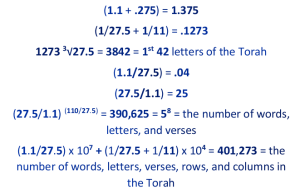The 401,273 elements in the Torah are a metaphor for the squaring of the circle whereby 400,000 is the area of the square that inscribes a circle with an area of 314218.4… or about 314220. This aligns with the small gematria of “I am that I am (אהיה־אשר־אהיה)” found at Exodus 3:14 and the numerical value of the 3 iterations of the Names Ehyeh (אהיה) and YHVH (יהוה), and with the 42-Letter Name divided by its powerful first line (3701/506) = 7.31422, which in turn aligns with the 6 different values that are found 26 separate times in the Torah—as in the numerical value of the YHVH (יהוה) —whose collective value is 3142, and it also aligns with the first 8 letters of the Torah (ברא־שיתבר) that have string gematria of (22131422).

### In the Matrix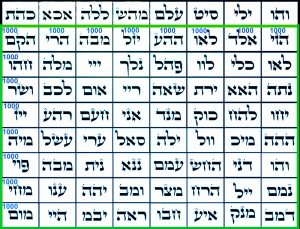The relationship between 11 or 1.1 and 27.5 extends deep into the 72 Triplet Matrix. As we know, astonishingly its first row of 8 Triplets totals 1143, out of the total 9143 value of the Matrix separating the bottom 8 rows and 8 columns into a square block of 8000, and moreover that 1143 is also the average of the 8 columns (9143/8) = 1143.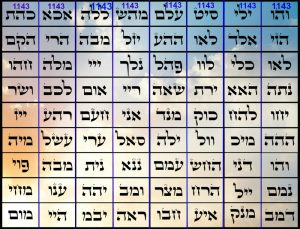There is though, a segment of 4 consecutive Names (ריי), (שאה), (ירת), (האא) leading up to the summit platform that also sum to 1143. These are the 26th, 27th, 28th, and 29th Triplets. While unbelievable, it gets better in that the average of these 4 is (1143/4) = (275 x 4) + (11 x 4) and the sum of those 4 ordinal positions (26 + 27 + 28 + 29) = 110, the major interval and integration of the two core cycles and frequencies or 110/4 = 27.5 and 110/10 = 11. It also means this purposeful design was meant to illustrate this connection to the radiant Source of the universe.### The Primal Frequency and the Circle that Turns Time

So, when the Primal Frequency of 27.5 Hz is applied, all numbers have a residue or binding connection to the number 9, except every 11th one, a cycle to which our Sun strictly adheres. This makes the 10 bundled numerical elements extraordinary, and it makes the exceptional unbound series of every 11th number extraordinary as well.

This is a rhythm. It is a regular heartbeat for both the Sun and mathematics.

If we were to take them as a series of 10 angles (9o, 18o, 27o, 36o, 45o, 54o, 63o, 72o, 81o, and 90o), they would sum to 495o or 1 full circle (360o) plus an additional 135o turning further around it. This is 1 full circle plus an additional 37.5% of a circle. Thus, the summary angle 495o of every bundle of 10 represents 1 and 37.5% circles or 137.5% of a circle, which is 1.375 circles, and that translates to 2.75π radians, and to 50 x 27.5 cubits.

The turning circle in increments of 9o occurs in two sequential segments (9o, 18o, 27o, 36o, 45o), which equals 135o, and (54o, 63o, 72o, 81o, and 90o), which equals 360o.  The first set of 5 angles equals 135o of one circle and the second set of 5 equals a second complete circle. The two sets of 5 angles each are 27.27% and 72.72% of the total 495o respectively. Alternatively, the 3 angles (36o, 45o, 54o) also equal 135o.

 Chochmah 0.363636 Binah 0.727272 Da’at 0.90909 Chesed 0.454545 Gevurah 0.818181 Tiferet 0.181818 Netzach 0.545454 Hod 0.090909 Yesod 0.272727 Malchut 0.636363Laid out in their sequential order those 3 angles (36o, 45o, 54o) that equal 135o correspond to the right column of the Tree-of-Life (Chochma-Chesed-Netzach) and their total remainder is 1.363…, an angle of 135o. Since Keter or the 11th dimension is separate, unbound, and indivisible and thus contains the whole number or integer values within the dynamic, it is not included in the central column. Thus, the central column (Da’at-Tiferet and Yesod-Malchut) of the Tree-of-life has the value (.9090 + .1818 + .2727 + .6363) = 2. While the left column (Binah-Gevurah-Hod) has a remainder value of 1.636 to complement the right column, making (1.363 + 1.636) = 3 for the two of them. Therefore, the left and central columns align to form one complete 360o circle and Keter aligns with the right column to form the upper circle, and this is a deep secret. The numbers and sefirot just automatically line up this way, cosmically designed as interlocking pieces in a puzzle, with the 4 sefirot central column forming a diameter within the circle of the 6 sefirot of the right and left columns.

Moreover, since 135o is 37.5% of a circle, the rest of the circle is (10037.5% = 62.5%, as in H’Keter (625) and as in the square root of all the words, letters, and verses in the Torah, 625, which is also (27.5/1.1)4.

Like the 2 cubes in the Tree of Life, the 2 circles or spheres are comprised of the 10 (angles, remainders, sefirot, or dimensions) of 137.5% and the 11th or Keter percentage or dimensioncomprises 62.5%.

Turning the circle by the Phi (φ) angle, 137.5o will create infinite parallel pathways around the circle, like the Hebrew word for parallel and for “to receive,” kabbalah of numerical value 137 which combined with 5 that represents Phi (φ).

Now, we are faced with a parallel concept that the sacred cubit or Primal Frequency applied to the circle will advance the circle by 2.75π radians at a time, which is 1.375% of the circle. This is the natural heartbeat or rhythm of the Spherical Time bubble, and it matches that of the Sun.

This also means that the Primal Frequency (27.5 Hz) turns the circle at the Phi (φ) angle. Conversely, the movement of the cosmic clock by 9o in cycles of 10, creates both the Phi (φ) angle at the Source of the infinite paths of Spherical Time and it creates the sacred cubit (27.5”), which is the resultant 2.75π radians divided by Pi (π).

### As the Clock Turns

With the primordial dynamics at work, the sum of the 10 resultant angles listed above, when each is divided by the Primal Frequency (27.5), equals 495o/27.5 = 18o, and the sum of their 10 radians is 3.14159

This is important. That extra 135o angle that wrapped around the circle is .375 or 37.5% of the circle, leaving a remainder of .625 of the 2nd circle, as in (20001375) = 625, which matches the dynamic splitting of the Alef-bet into the 11 Odd/Even letters.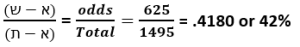It also matches the standard gematria value of the entire Torah divided by the full sofit value that is (58002 + 625) or 21009826/33640626 = .625, approximately 62.5% and their difference is 12630800/33640626 = .375, approximately 37.5%.

Yet another connection to Phi (φ) and the Alef-bet is found in the figure .4180 in that the sum of the first 17 Fibonacci Numbers is 4180. It is also amazing that the Alef-bet is comprised of natural splits into 625 and 495 and 1000 as in the contained encircling of Phi (φ).

This intersection of the Torah, the Tree-of-life, and the radiance of Phi (φ) is almost to be expected at this point, which in no way demotes its significance or the reasoning behind structuring the universe this way.

These particular and extraordinary dynamics are not why we said is important though. The value 1375” equals 50 cubits, the height of each of the 10 Gates of the Future Holy Temple, but that is not why it is important either. And while 1375” or 50 cubits is also the height of Noach’s Ark, the volume of that Ark is 45,000 cu3, which may connect it to Man (45) and to the angle 495o that equals 11 x 45, creating an interesting analogy to the 11-year Sun cycles and the 11-bundled cycles of the Primal Frequency residues; nevertheless, this is not why it is important either.

What is significant is that the Phi (φ) angle is 137.5o, and that it is 5 x 27.5 Hz.

As the Phi (φ) angle (137.5o) continually proceeds around a 360o circle, it will generate the Phi (φ) spiral, etching a different place-marker on Spherical Time with every turn, indefinitely.  On a single rotation cycle there will be two turns of (137.5o) or 275o within every circle and a remainder of (360o2(137.5o)) = 85o.  The ratio of the full circle to 85o is 360/85 = φ3.Those two first turns sum to (137.5o + 137.5o) = 275o, giving us the 27.5” sacred cubit and the Primal Frequency. So, a cubit is defined as 1” for every 10o in 2 x Phi (137.5o) turns, and the frequency 27.5 Hz would be 1/10th the 2 x Phi (137.5o) turns.

You could not use that physically unless you are building a circle with a 57.295779” radius, but it is significant metaphysically. It is significant in Binah hyperspace. It is significant to the vibrational frequency of 27.5 Hz. The connection to Phi (φ) and Pi (π) through the circle is included in every cubit measured out and used in Holy construction, or in divinely inspired construction. It is at the core of Spherical Time.

Each one of the Phi (φ) angle (137.5o) turns is thus equal to 5 equal parts of 27.5o each.

Each one of the Phi (φ) angles (137.5o) of 5 equal parts 27.5o is equal to 1/φ2 as a percentage of the circle, so both Phi (φ) angles (137.5o) or 10 equal parts 27.5o is equal to 2/φ2 as a percentage of the circle. Its’ inverse is approximately 1.30, aligning it with the 13o shift that brings the Alef-bet Odd/Even split into perfect alignment with the Phi (φ) proportion. This is equivalent to a 54-integer shift, as in the Inflection Point of the quotient (27.5) scale.  This only happens if they are cosmically related through hyperdimensional mathematics, connected through a significant node in a hyperdimensional network.

That inverse of the 2 Phi (φ) angles (137.5o) as a percentage of a circle is 2/φ2 = 1.30909, which is also precisely equivalent to the sum of the first 8 integers in quotient (27.5) out of the first 10 that equal 2 at the first interval.

 1 0.036364 0.036364 2 0.072727 0.109091 3 0.109091 0.218182 4 0.145455 0.363636 5 0.181818 0.545455 6 0.218182 0.763636 7 0.254545 1.018182 8 0.290909 1.309091 9 0.327273 10 0.363636 55 2

This only happens if the Alef-bet and the numeric properties of the universe are in perfect alignment and that only happens if they have the same Creator.

That figure of the Phi cubed (φ3) percentage residue or 85o remainder after 2 Phi (φ) angle (137.5o) twists in the circle has a special relationship to the Spherical Time bubble in that φ3/√φ = 3.330….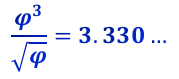It is the same 3330 years that lie between the reception of the Torah at Mt Sinai of numerical value 130 in 2448 HC and the event horizon in 5778 HC, 66.6 jubilee years later, as in 2448/5778 = φ3, which is approximately 4.24, as in Moshiach Ben David, Cosmic Consciousness. And we should not forget that 2448 HC is 1313 BCE, as in the x1.313 scale of the Alef-bet.

This only happens if the Calendar of our History and Torah and the numeric properties of the universe and the Alef-bet are in perfect alignment, and that only happens if they have the same Creator.

Of course, the fact that the Sun’s rotation at the equator is approximately 24.48 days and 5778 is φ18 must play a role as well with Phi (φ) at the core of the Spherical Time Clock and 5778 at its extremity. As we know, the Sun’s cycle ties in with the clock and 5778 is the surface temperature of the Sun, 5778 K, the same Sun whose longitudinal rotation is 27.275 days, known as the Carrington synodic rotation, like the 27.3 day monthly sunspot cycle and the 27.3 day lunar rotation and orbit.

Moreover, as we saw in the previous article about the Divine Simulation, the ratio of the Sun’s mass to that of the Earth is 333,000 and the Earth orbits the Sun at 66,600 mph.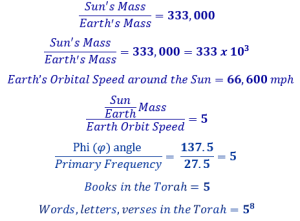This only happens if our Solar System and the numeric properties of the universe are in perfect alignment, and that only happens if they have the same Creator.### An Abstract Way of Seeing the Two Primal Frequencies

To most of the world what we have been studying is extremely abstract already, yet abstractions are what open our eyes. The more we stay tied to the physical and the concrete the less we understand.  We can break down the 27.5 Hz Primal Frequency into two parts and thus incorporate the other Primal Frequency 1.1 Hz into it with the equations 1.1(1 + 1.5) = 2.75 and 1.1(10 + 15) = 27.5. If it makes it easier to visualize why we are breaking up 2.5 or 25 into two components think of it as 1.1(a + b) = 27.5 and you can see that 25 may indeed be more that a single solitary number.

Indeed, 15 is (יה) and 10 is (י), and 1 is (א) and 1.1 and/or 11 is (וה), so 1.1(a + b) = 27.5 can be understood as (וה)*((יה) + (י)) = (וה)*(יה) + (וה)*(י) = 27.5 or (אי)*((יה) + (י))  = 2.75 from the YHVH (יהוה) and from Ehyeh (אהיה).

Since we still do not comprehend those cosmic fields properly, let us return to the numbers and the equation 1.1(1 + 1.5) = 2.75, and if instead we multiply them, (1.1*1) and (1.1*1.5), and then take the square roots of their products we get √(1.1*1) + √(1.1*1.5) = 2.33333210.

The ratio of the sacred cubit to the foot, or the Primal Frequency to the clock of 12 is 27.5/12 = 2.291666 and (2.333332102.291666) = .041666 or about .042. Nevertheless, the ratio of the height of the Tower of Truth, 210 sacred cubits, to the circle is 210/360 = .58333, or (1 – .41666). Moreover, the average letter value of the 64 Triplets that form the 8000-value foundation square, the 203 Foundation Cube/Stone within the 72 Triplets is exactly (8000/64/3) = 41.666. Furthermore, 1/.041666 = 24.00032, as in the 24 hours in a day.

### The Heartbeat of the YHVH (יהוה).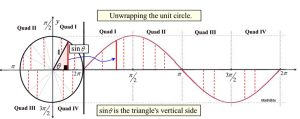When we hear frequency we should picture sine waves, a number of crests or pulses in a single unit. The two frequencies that resonate with the number 9, run side by side, 27.5 pulses and 1.1 pulses.

Like all wave patterns, they are generated from the circle, or the geometric encircling of a central point to form sine waves. The closeness of the peaks in those wave patterns determines their frequency. And a wave of 27.5 Hz means that there are 27.5 wave crests or pulses every second. Every 4 cycles of 27.5 Hz bring the two Primal Frequencies (27.5 Hz and 1.1 Hz) into alignment. This means that every 4 seconds, the 10 wave crests of 1.1 Hz align with the 110 crests or pulses of 27.5 Hz. This goes back to the basic equation that the sum of the integers from (14) = 10, in other words the sum of the packets of signals (1 + 2 + 3 + 4) = 10.

The 1.1 Hz wave is 25 times longer (from crest to crest) than the 27.5 Hz one so the 2 of them are out of sync, except on the longer scale when they periodically line up or crest at the exact same moment, which is every 2 bursts of 27.5 pulses for every 50 bursts of 1.1.  Mathematically that works out to (2 x 27.5) = (50 x 1.1) = 55. Waves are additive, so at this point their wave height is at its maximum, twice its normal crest, like the solar maximum every 11 years, and the galactic maximum every 12,000 or 11,556 years.

Both Primal Frequencies beat every 11 integers and sync up every 5 groups or cycles of those beats. What does this mean?

 1 0.909091 2 1.81818 3 2.72727 4 3.63636 5 4.54545 6 5.45455 7 6.36364 8 7.27273 9 8.18182 10 9.09091 11 10 12 10.9091 13 11.8182 14 12.7273 15 13.6364 16 14.5455 17 15.4545 18 16.3636 19 17.2727 20 18.1818 21 19.0909 22 20

As we divide by 1.1, the results get incrementally closer to a whole number every 11th integer, this is one beat. Those whole numbers are in increments of 10. As we divide by 27.5, the results get incrementally closer to a whole number every 55th integer; this is two pulses. Those whole numbers are in increments of 2. Every 11 integers the results get incrementally closer to a whole number when multiplied by 10; this is one beat. Those whole numbers are in increments of .4, so when multiplied by 10 they give us 40.

 1 0.036364 0.909091 2 0.072727 1.81818 3 0.109091 2.72727 4 0.145455 3.63636 5 0.181818 4.54545 6 0.218182 5.45455 7 0.254545 6.36364 8 0.290909 7.27273 9 0.327273 8.18182 10 0.363636 9.09091 11 0.4 10 12 0.436364 10.9091 13 0.472727 11.8182 14 0.509091 12.7273 15 0.545455 13.6364 16 0.581818 14.5455 17 0.618182 15.4545 18 0.654545 16.3636 19 0.690909 17.2727 20 0.727273 18.1818 21 0.763636 19.0909 22 0.8 20 44 1.6 40 45 1.63636 40.9091 46 1.67273 41.8182 47 1.70909 42.7273 48 1.74545 43.6364 49 1.78182 44.5455 50 1.81818 45.4545 51 1.85455 46.3636 52 1.89091 47.2727 53 1.92727 48.1818 54 1.96364 49.0909 55 2 50

Therefore, while the heart beats every 11 integers on both of the frequency scales, 5 simultaneous beats each in every 55 integers, there is only one whole number pulse (2) of the 27.5 Hz Frequency for every 55 integers verses 5 whole number pulses (1020304050) for the 1.1 Hz Frequency. That is a frequency to frequency ratio of 5 wave crests for every 1, and the average of the 2 Primal Frequencies for those 55 integers or 5 beats is (50 + 2)/2 = 52/2 = 26, as in the value of the YHVH (יהוה).

The individual heartbeats are the intervals, while the pulse beats every 5 intervals for a total of 55 sub-beats. While these 55 sub-beats are baked into the nature of the basic numbering system aligned specifically to the sum of the integers from (110) = 55, cosmologically 55 is not a coincidental number or causational number. It is the basic unit in our time, and the value of the two feminine aspects (הה) of the YHVH (יהוה) in an energetic sense.

### The Heartbeat of Time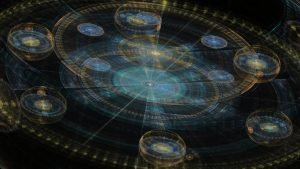Meanwhile the sum of the first 5 intervals (heartbeats) of the 27.5 Hz Frequency and the sum of the first 5 intervals (heartbeats) of the 27.5 Hz Frequency equal (.4 + .8 + 1.2 + 1.6 + 2) + (10 + 20 + 30 + 40 + 50) = (6 + 150) = 156, as in Joseph (156), whose 6 dreams were presented in pairs of 2. The 5 intervals (heartbeats) over both Frequencies covers a total of (2 x 55) = 110 integer points or quotients, as in Joseph’s age, 110 years. This is both a cosmic alignment and a cosmic breadcrumb. There is also a resonance with the Torah whose ratio of its total sofit value to its standard value (32775966 /21009826) is 1.56000.

The analogy deepens as Joseph’s 2 personal dreams were about a clock face with 12 subordinate elements equidistantly notched around a circle. When we dividethe Spherical Time circumference by 12, each hour is 3025 years apart or 55.002 years apart.  This is an important element to the dynamic of Spherical Time, and matches the 3025’ perimeter of the Pyramid of the Sun.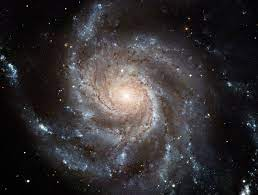Dividing by Pi (π), each 3025-year arc or hour is 963 earth years of the Event Horizon diameter. Each of those 12 arcs of 963 years is equal to the sum of the first 24 Prime Numbers, 963.

While Adam, who lived 930 years, was 33 years shy of 1 arc or 963 years, the Flood occurred after 1 year or arc of 963 years plus an additional 693 years, which is 72% of an arc, or 270 years shy of 2 arcs.

### A Divine Inflection Point

Another inflection point is found at integer 715. At precisely 65 pulses of the Cosmic Heart, as in the value (65) of Adonai (אדני), we find that the Primal Frequency 27.5 Hz yields a quotient of exactly 26, the value of Adonai’s (אדני) complementary counterpart, the YHVH (יהוה).

The difference between Adonai (אדני) and the YHVH (יהוה) is numerically (6526) = 39, as in the value of “G-d is One (יהוה־אחד)” from the Shema and thus from the Torah. Meanwhile, the 39th Triplet (רהע) of the 72 Triplets has the 275, as in the Primal Frequency. And the sum of the integers from 26 to 65 is 1820, as in the 1820 YHVH (יהוה) in the Torah and the Path of One.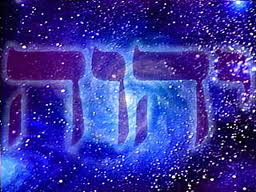And given this cosmic and profound relationship, it is incredible that integer 715 also yields the quotient for the other Primal Frequency (1.1 Hz) of 650 or 10 times the value of Adonai (אדני). Most astonishing, though, is that together they give us (26 + 650) = 676, which is 262. And (715676) = 39.

This is 65 heartbeats (intervals) and 650 sub-beats for each Frequency, or 130 beats and 1300 sub-beats for both together, as in Mt Sinai (the ladder) of numerical value 130, which is astounding because the 1820 YHVH (יהוה) in the Torah times the 715 Divine inflection point is (1820 x 715) = 1301300 that also reflects Love, Ahava (אהבה) and Oneness (אחד) or 13 and 13, like the year the Torah was given to Man (1313 BCE and the exponent of the Alef-bet (x1.313).

How amazing is it that so deep into the interaction of the Primal Frequencies the forces of the universe are designed so that the YHVH (יהוה) and Adonai (אדני) would come together in such a special and unique way along with the Path of One and be interconnected with the 72 Triplets, the 15 Triplets of the Shema the design of the Torah, history, our calendars, and the Alef-bet.   And it was all hidden away in a number whose only claim to fame is that it is half (715/2) = 358, Moshiach.

 704 25.6 640 705 25.6364 640.909 706 25.6727 641.818 707 25.7091 642.727 708 25.7455 643.636 709 25.7818 644.545 710 25.8182 645.455 711 25.8545 646.364 712 25.8909 647.273 713 25.9273 648.182 714 25.9636 649.091 715 26 650 676 716 26.0364 650.909 717 26.0727 651.818 718 26.1091 652.727 719 26.1455 653.636 720 26.1818 654.545

This is indeed an inflection point because the combined results get closer to an exact square of the 27.5 Primal Frequency quotient until we reach 26, and then they get further and further away from it afterwards. This not only makes it unique in its metaphysical significance but mathematically as well.

It is a singular confluence in the fields, with the 1.1 Hz Primal Frequency being associated with the field and power of Adonai (אדני) and the 27.5 Hz Primal Frequency with that of the YHVH (יהוה), two specific Cosmic forces eternally entwined, as we saw with Pi (π) and Phi (φ) within the 42-Letter Name. This time, as they are combined, they release the power of the Light through squaring (262), as Rabbi Chaim Vital of blessed memory advised centuries ago.

We could even say that these are the frequencies of the YHVH (יהוה). This is most significant, perhaps most of all.  The sum of the results for any integer divided by 27.5 and 1.1 and then divided by the 27.5 result will always be 26.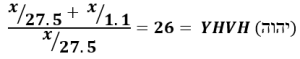### An Understanding of Time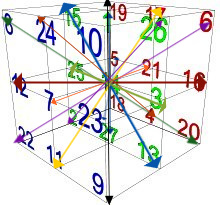We now have a better understanding of how the 26 vectors of the Essential Cube of Creation are connected to the Primal Frequencies of the aether and the Cosmos, and why applying the matrices to selectively rotate and change them is so important. As we saw, Spherical Time is a sphere or torus with a set circumference, 36304.24470 years, and its radius (5778 years) is a vector. As a clock those vectors can be rotated in 12 equidistant arcs, and yet the Essential Matrices are cable of rotating them in any direction and alter their length to any point within the Event Horizon, thus altering what we call time, and what we understand as the time-paths.  This is a critical understanding. Time is linear only in our minds.

### The Source is One

Another interesting confluence or phase happens at the 1000th integer, representing Binah. The cumulative value of all the 27.5 Primal Frequency quotients is exactly 18200, as in Jacob (182) and as in the 1820 YHVH (יהוה) in the Torah. It is even more primally associated with the sum of the 26 squares and 26 cubes of the 26 radial vectors for the 27 letters within the normal Magic Essential Cube of Creation, (1638 + 16562) = 18200.

As previously explained those 1820 YHVH (יהוה) and the 18200 sum of the 26 Essential vector distances are directly connected to the Path of One, the sequential summation of the series of order of 13, that begins with Echad (אחד), One (113914551820), including the value 91 that is reflected in the 715 Divine inflection point, the 65 pulses and the resultant 26, or (65 + 26) = 91, Adonai (אדני) and YHVH (יהוה).

Moreover, as noted, 1820 is part of the Path of One or the order of 13 that beginnings with Echad (אחד), One: 113914551820 and that is built into the Torah and is at the heart of the super network of the Cosmos. So, it is even more astonishing that the cumulative value of all the 1.1 Primal Frequency quotients through that same 1000th integer is exactly 455,000, as in the value (455) of the 3 Aspects of Ehyeh (אהיה) in Binah times 1000, another appellation for the hyperspace dimension of Binah. This is why we find 455 and 1820 side by side in Pascal’s triangle.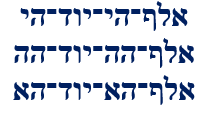While the 1000th Triangular Number is 500500, the frequency of the 1820 YHVH (יהוה) is exactly 1/10th that or (1820 x 27.5 Hz) = 50050, and when divided by 1.1 Hz, it equals 45500. The Source is One.

If that were not enough of a cosmic synchronicity, the value of that 1000th integer quotient for the 27.5 Primal Frequency is 36.36363, as in the value of H’Moshiach (363) the Cosmic Consciousness. Moreover, the value of the 1000th integer quotient for the 1.1 Primal Frequency is 909.090, as in the value of Melech (מלך), King (90), whose ordinal value is 36, giving it a complete value of (90 + 36) = 126, as in the 126-value of every face, plane, and of the 3 axes that make up the normal Magic Essential Cube of Creation.  It hints at King H’Moshiach.

 990 36 900 17838 445950 991 36.0364 900.909 17874 446851 992 36.0727 901.818 17910.1 447753 993 36.1091 902.727 17946.2 448656 994 36.1454 903.636 17982.4 449559 995 36.1818 904.545 18018.5 450464 996 36.2182 905.455 18054.8 451369 997 36.2546 906.364 18091 452276 998 36.2909 907.273 18127.3 453183 999 36.3273 908.182 18163.6 454091 1000 36.3636 909.091 18200 455000 1001 36.4 910 18236.4 455910

### Phase Points

The phase point of the juxtaposition of the two Primal Frequencies is where both waves are cresting or in phase at the same moment. This occurs whenever their resultant values are both whole numbers. It occurs every 55 integers like a perfect ladder through time. Each one of the phase levels is 5 pulses of 11 sub-beats or 55 higher than the next.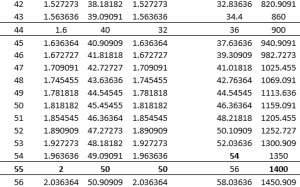While 55 is the first of the known phase points of the two scales/cycles, the cumulative sum through the 55th integer of the 1.1 Hz scale is exactly 1400, as in position 14 at the center of the same Magical Essential Cube of Creation. What deepens this connection is that 55/1.1 = 50, the value of the Hebrew letter Nun (נ) that corresponds to that 14th position.

 98 3.563636 89.09091 176.4 4410 4586.4 99 3.6 90 180 4500 4680 100 3.636364 90.90909 183.6364 4590.909 4774.545 101 3.672727 91.81818 187.3091 4682.727 4870.036 102 3.709091 92.72727 191.0182 4775.455 4966.473 103 3.745455 93.63636 194.7636 4869.091 5063.855 104 3.781818 94.54545 198.5455 4963.636 5162.182 105 3.818182 95.45455 202.3636 5059.091 5261.455 106 3.854545 96.36364 206.2182 5155.455 5361.673 107 3.890909 97.27273 210.1091 5252.727 5462.836 108 3.927273 98.18182 214.0364 5350.909 5564.945 109 3.963636 99.09091 218 5450 5668 110 4 100 222 5550 5772 111 4.036364 100.9091 226.0364 5650.909 5876.945

At the 10th pulse of those 11 beats, corresponding to integer 110, the cumulative total for the 27.5 Hz scale is 222 and it is 5550 for the 1.1 Hz scale, or 5772 cumulatively for both. This is the second phase point. While 222 = (60005778), the ratio (222/1.1) = 201.8, yet another cosmic linkage between 5778 HC and 2018 CE built into the mathematical backbone of our universe. It is appropriate that this has to do with the phasing of the Primal Frequencies or heart beats of our universe, especially since the value 5772 is Euler’s Constant (.5772156…).

Whether counting integers or years or leaves on a tree, the effect and symmetry and connection to the core radiation of the universe is the same.

 153 5.56364 139.091 428.4 10710 154 5.6 140 434 10850 155 5.63636 140.909 439.636 10990.9 156 5.67273 141.818 445.309 11132.7 157 5.70909 142.727 451.018 11275.5 158 5.74545 143.636 456.764 11419.1 159 5.78182 144.546 462.546 11563.6 160 5.81818 145.454 468.364 11709.1 161 5.85454 146.364 474.218 11855.5 162 5.89091 147.273 480.109 12002.7 163 5.92727 148.182 486.036 12150.9 164 5.96364 149.091 492 12300 165 6 150 156 498 12450 166 6.03636 150.909 504.036 12600.9

Joseph lived for 110 years, representing the second phase point, or rung on the cosmic ladder, while the sum of the values of both scales together for the 165th integer, the third rung or phase point, is 156, the numerical value of Joseph’s name. Of note also is that the third phase point or 165 is the base of the Tower of Truth Triangle.

 172 6.25455 156.364 541.018 13525.5 173 6.29091 157.273 547.309 13682.7 174 6.32727 158.182 553.636 13840.9 175 6.36364 159.091 560 14000 176 6.4 160 566.4 14160 177 6.43636 160.909 572.836 14320.9 178 6.47273 161.818 579.309 14482.7 179 6.50909 162.727 585.818 14645.5 180 6.54545 163.636 592.364 14809.1

Abraham lived 175 years and the cumulative sum of the 1.1 Hz scale is 14000 for the 175th integer, again as in the position 14 at the center of the same Magical Essential Cube of Creation.

 264 9.6 240 1272 31800 33072 265 9.63636 240.909 1281.64 32040.9 33322.6 266 9.67273 241.818 1291.31 32282.7 33574 267 9.70909 242.727 1301.02 32525.5 33826.5 268 9.74545 243.636 1310.76 32769.1 34079.8 269 9.78182 244.546 1320.55 33013.6 34334.2 270 9.81818 245.454 1330.36 33259.1 34589.4 271 9.85454 246.364 1340.22 33505.4 34845.7 272 9.89091 247.273 1350.11 33752.7 35102.8 273 9.92727 248.182 1360.04 34000.9 35360.9 274 9.96364 249.091 1370 34250 35620 275 10 250 260 1380 34500 35880 276 10.0364 250.909 1390.04 34750.9 36140.9 277 10.0727 251.818 1400.11 35002.7 36402.8 278 10.1091 252.727 1410.22 35255.4 36665.7

Then 100 integers later, a very odd thing happens when we get to the cumulative sum of all the integers through 275, the 5th phase point.  The 1.1 Hz scale sums to exactly 34500, as in Moses (345) and the 5th (מהש) of the 72 Triplet, while the 27.5 Hz scale sums to 260, as in the YHVH (יהוה) and the 8 x 8 normal magic square constant.  Meanwhile, the cumulative sum of both scales totals (34500 + 1380) = 35880, as in Moshiach and Moses’s age (80) at Mt Sinai, which in turn aligns with the 80 times the numerical value of Moshiach, 358, is utilized in the Torah. And 1380 may be metaphysically connected with the 1380th YHVH (יהוה) in the Torah found in the first verse of the Shema at Devarim 6:4 which conceals the set of 15 Triplets based on the 42 Letters of the YHVH (יהוה). This is the 441st YHVH (יהוה) until the end of the Torah, as in emet, truth.

As for the 620th integer, that describes keter, the crowning dimension, the cumulative frequency (27.5 Hz) values of all 620 integers are 7000.363, as in H’Moshiach and the 7th millennium or Great Shabbat. Meanwhile, since the cumulative frequency (1.1 Hz) values of all 620 integers are 175009.18, the total cumulative frequency values for both Primal Frequencies is 182009.54 = (182000 + 9.54), representing Abraham’s age (175); Jacob (182); the digital position #954 of the final …5778… in Pi (π); and the 18200 sum of the 26 Essential vector distances that are directly connected to the Path of One and 1820 YHVH (יהוה). Thus, at the frequency of Keter, several key concepts are linked together in a super network node.

Part 4 follows shortly where we see just how much of our physical world is defined by these primordial field interactions and frequencies, especially our DNA.